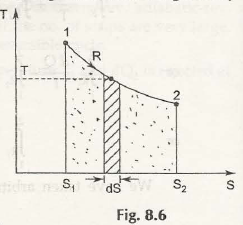Consider this figure:From there we get:

dS = d QR / T

d QR = T dS

QR = 2ʃ1 T Ds

= area under the curve 1 – 2

= Heat transfer in the process.

• In x-axis we take entropy (s) and in y-axis we take Kelvin temperature (T).
• This is basically known as called T – 5 diagram.
• T 1 – 2 is a reversible process.